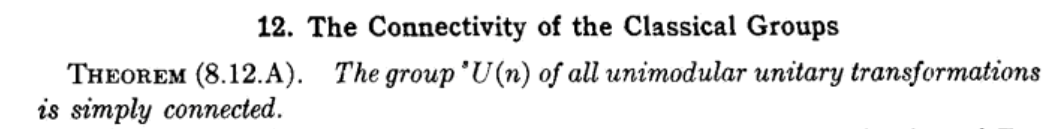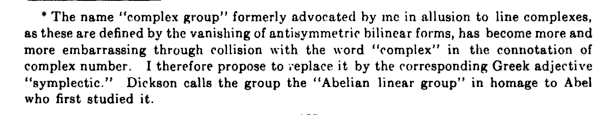# Who originated the standard symbols for Lie groups GL, SL, SU, etc.?

Who was first to use symbols GL, SL, O, SO, U, SU, Sp and their projective versions, and how did this notation become standard?

The notation appears in fairly modern form in Weyl's "The Classical Groups" except that he uses $O^+$ for SO. I know that Weyl coined the term "symplectic group", so the notation Sp certainly originates with him.

Can anyone confirm or deny that Weyl was the originator of the standard symbols for Lie groups?

Thanks.

• Interesting. I hadn't seen $O^+$ before, but it seems more natural and I wish it had stuck. – Nate Eldredge Aug 22 '14 at 22:46
• @NateEldredge: Why do you consider $O^+$ to be more natural than SO as notation for what is (away from char. 2) exactly ${\rm{O}} \cap {\rm{SL}}$? The SO convention adapts nicely to variants like SU and even to Pin groups from Spin groups. – user54268 Aug 23 '14 at 2:29
• I've used $O^+$ when I also need the complement $O^-$. Is there a more natural way to denote the set of orthogonal matrices with determinant $-1$? – Carlo Beenakker Aug 23 '14 at 8:49

It's hard to provide definitive confirmation of Weyl's role, but his 1939 book was highly influential in all further developments. It's important to realize that notation (and terminology) in mathematics tend to evolve over time, and what are now considered "standard" symbols for the various linear/Lie groups didn't all spring up at once. Pioneers like E. Cartan made their own choices, of course, many of which have been modified (such as the conventions for denoting Lie algebras, originally called "infinitesimal groups").

Working over a variety of fields and other rings has strongly influenced the choice of symbols. Weyl himself tended to work just over $\mathbb{R}$ or $\mathbb{C}$. It's instructive to look back at the book Linear Groups by L.E. Dickson, published around 1900 and later reprinted in a Dover edition. His emphasis was on linear groups over finite fields ("Galois fields"), denoted by him GF[$p^n$]. Since he was aiming in particular at finite simple groups, he wrote LF for a "linear fractional" group, now usually denoted PSL. He and his contemporaries also used the term "homogeneous" a lot, which shows up in notations like HO ("homogeneous orthogonal") and GLH ("general linear homogeneous") or SLH.

Dieudonne's work on classical groups, emphasizing their structure and automorphisms, followed Weyl's by a decade or more. His need to work over much more general fields, and to emphasize the role of a chosen bilinear form, led to more elaborate notation than Weyl's, and he added $\Omega$ to $O$.

As you've pointed out, Weyl used O$^+$ rather than SO, which reinforces my point above: notation evolves over time. But the influence of his book made the earlier choices of Dickson and others far less standard. I'm unaware of any papers or books in the intermediate decades that did as much to shape the modern choices.

In his 1939 book Weyl indeed did not use the notation SO but $$O^+$$, and called it the proper orthogonal group, but he did use the notation SU, or more precisely $$^s$$U with a tiny superscript s.The name "special unitary group" does not appear, it is always "unimodular".

The footnote on the name "symplectic group", from the same book, is amusing: